# Measuring the Interaction Force Between a Droplet and a Super-hydrophobic Substrate by the Optical Lever Method

Engineering

## Your institution must subscribe to JoVE's Engineering section to access this content.

### Summary

The protocol aims to investigate the interaction between droplets and super-hydrophobic substrates in the air. This includes calibrating the measurement system and measuring the interaction force at super-hydrophobic substrates with different grid fractions.

Zhuang, S., Zhao, M., Wang, Z., Zhang, L., Huang, Y., Zheng, Y. Measuring the Interaction Force Between a Droplet and a Super-hydrophobic Substrate by the Optical Lever Method. J. Vis. Exp. (148), e59539, doi:10.3791/59539 (2019).

### Abstract

The goal of this paper is to investigate the interaction force between droplets and super-hydrophobic substrates in the air. A measurement system based on an optical lever method is designed. A millimetric cantilever is used as a force sensitive component in the measurement system. Firstly, the force sensitivity of the optical lever is calibrated using electrostatic force, which is the critical step in measuring interaction force. Secondly, three super-hydrophobic substrates with different grid fractions are prepared with nanoparticles and copper grids. Finally, the interaction forces between droplets and super-hydrophobic substrates with different grid fractions are measured by the system. This method can be used to measure the force on the scale of sub-micronewton with a resolution on the scale of nanonewton. The in-depth study of the contact process of droplets and super-hydrophobic structures can help to improve the production efficiency in coating, film and printing. The force measurement system designed in this paper can also be used in other fields of microforce measurement.

### Introduction

The contact between a droplet and a super-hydrophobic surface is very common in daily life and industrial production: water droplets sliding from the surface of lotus leaf1,2, and a water strider traveling rapidly over the water3,4,5,6. A super-hydrophobic coating on the exterior surface of a ship can help reduce the corrosion degree of the ship and reduce the resistance of the navigation7,8,9,10. There is great value for industrial production and bionics research in studying the contact process between a droplet and a super-hydrophobic surface.

To observe the spreading process of droplets on a solid surface, Biance used a high-speed camera to photograph the contact process and found that the duration of the inertial regime is mainly fixed by the drop size11. Eddi photographed the contact process between the droplet and the transparent plate from the bottom and side using a high-speed camera, which comprehensively revealed the variation of the contact radius of the viscous droplet with time12. Paulsen combined an electrical method with high-speed camera observation, thus reducing the response time to 10 ns13,14.

Atomic force microscopy (AFM) has also been used to measure the interaction force between the droplet/bubble and solid surfaces. Vakarelski used an AFM cantilever to measure the interaction forces between two small bubbles (approximately 80-140 μm) in aqueous solution during controlled collisions on the scale of micrometers to nanometers15. Shi used a combination of AFM and reflection interference contrast microscopy (RICM) to simultaneously measure the interaction force and the spatiotemporal evolution of the thin water film between an air bubble and mica surfaces of different hydrophobicity16,17.

However, since commercial cantilevers used in AFM are too small, the laser spot irradiated on the cantilever would be submerged by droplets or bubbles. The AFM has difficulties in measuring the interaction force between droplets and droplets/substrates in the air.

In this paper, a measurement system based on an optical lever method is designed to measure the interaction force between droplets and super-hydrophobic substrates. The force sensitivity of the optical lever (SOL) is calibrated by electrostatic force18, and then the interaction forces between droplets and different super-hydrophobic substrates are measured by the measurement system.

The schematic diagram of the measurement system is shown in Figure 1. The laser and position sensitive detector (PSD) constitute the optical lever system. A millimetric silicon cantilever is used as a sensitive component in the system. The substrate is fixed on the nanopositioning z-stage, which can move in vertical direction. When the substrate approaches the droplet, the interaction force causes the cantilever to bend. Thus, the position of the laser spot on PSD will change, and the output voltage of PSD will change. The output voltage of PSD Vp is proportional to the interaction force Fi, as shown in Eq. (1).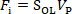(1)

In order to acquire the interaction force, SOL must be calibrated first. The electrostatic force is used as the standard force in the calibration of SOL. As shown in Figure 2, the cantilever and the electrode make up a parallel plate capacitor, which could generate electrostatic force in a vertical direction. The electrostatic force Fes is determined by the voltage of the DC power supply Vs, as shown in Eq. (2)19,20,21.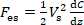(2)

where C is the capacitance of the parallel plate capacitor, z is the displacement of the cantilever free end, and dC/dz is called capacitance gradient. The capacitance could be measured by the capacitance bridge. The mathematical relationship between C and z can be fitted by a quadratic polynomial, as shown in Eq. (3).(3)

where Q, P and CT are the coefficients of the quadratic term, the primary term and the constant term respectively. Therefore, the electrostatic force Fes can be expressed as Eq. (4).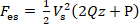(4)

Since the overlap area of two plates of the capacitor is very small, the elastic force acted on the cantilever can be expressed as Eq. (5), according to Hooke's law: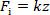(5)

where k is the stiffness of the cantilever.

When the elastic force and electrostatic force applied on the cantilever are equal (i.e., Fi = Fes), the cantilever is in equilibrium. Eq. (6) can be derived from Eqs. (1), (2) and (5):(6)

In order to reduce the uncertainty of calibration results, a difference method is used to calculate SOL. The results of two experiments are taken as Vs1, Vp1 and Vs2, Vp2, and are substituted into Eq. (6):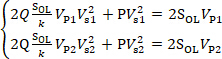(7)

Transforming the equations and subtracting the lower equation from the upper equation in Eq. (7), the parameters Q and k are eliminated. Then the calibration formula of SOL is obtained, as shown in Eq. (8):(8)

Performing a series of experiments, the curve is drawn with P(1/Vp1-1/Vp2) as the ordinate and 2(1/Vs12-1/Vs22) as the abscissa. The slope of the curve is SOL.

After obtaining SOL, the electrode will be replaced by different super-hydrophobic substrates. The interaction forces between droplets and super-hydrophobic substrates will be measured by the system shown in Figure 1.

### Protocol

1. Assembly of the SOL calibration system

1. Assemble the SOL calibration system according to the schematic diagram shown in Figure 2.
2. Fix the laser to a support, making the angle between the laser and the horizontal direction be 45°.
3. Fix the PSD to another support, making the PSD perpendicular to the laser. Connect the PSD to the data acquisition device and the data acquisition device to the computer.
NOTE: These angles are determined by the experimenter's visual measurement and need not be exactly 45° or 90°.
4. Fix the wider end of the cantilever to a holding device while the other end is suspended. Fix the holding device to a two-dimensional high-precision displacement stage.
NOTE: The dimensions of the cantilever are shown in Figure 3.
5. Fix the plate electrode to the nanopositioning z-stage by a clamping device.
NOTE: The nanopositioning z-stage can bring the electrode to move along the z-axis with a displacement resolution of 1 nm.
6. Connect the positive pole of the capacitive bridge with the cantilever and the negative pole with the plate electrode.
7. Install a high-speed camera, whose line of sight is perpendicular to the cantilever.
8. Adjust the position of the plate electrode, making the vertical distance between the plate electrode and the cantilever be about 100 μm, and the overlap length about 0.5 mm.
NOTE: These distances are checked by image processing.

2. Measurement of the capacitance gradient

1. Use the computer to control the capacitance bridge to collect the capacitance changes between the plate electrode and the cantilever in real time. Set the sampling rate to 0.5 Hz.
2. Control the nanopositioning z-stage by computer to drive the plate electrode to step upward with a step of 10 μm and a step number of 6 and stay for 10 s after each movement.
3. Change the movement direction of the plate electrode to downward, and repeat step 2.2.
4. Determine the relationship between the capacitance and displacement of the plate electrode in the measurement result, and obtain the value of P according to the Eq. (3).
5. Repeat steps 2.1–2.4 5x and calculate the average value of P.

3. Calibration of the optical lever

1. Disconnect the connection between the capacitive bridge and the cantilever/plate electrode.
2. Connect the positive pole of the DC power supply with the cantilever and the negative pole with the plate electrode.
3. Adjust the relative position between the laser, PSD and cantilever to make the laser reflected on PSD by cantilever.
NOTE: The laser spot is a circle about 2 mm in diameter.
4. Control the DC power supply by computer to apply voltage varying with time on the parallel plate capacitor. At the same time, collect the output voltage of PSD in real time by the data acquisition device.
1. Set the sampling rate of the data acquisition device to 1,000 Hz.
2. Set the initial voltage of the DC power supply to 0 V and hold for 5 s.
3. Increase the voltage by 25 V and hold for 5 s.
4. Repeat step 3.4.3 4x until the voltage increases to 125 V.
5. Decrease the voltage by 25 V and hold for 5 s.
6. Repeat step 3.4.5 4x until the voltage decreases to 0 V.
5. Determine the relationship about the output voltage of PSD and the DC supply voltage in the measurement result, and obtain the value of SOL according to Eq. (8).
6. Repeat steps 3.4–3.5 5x and calculate the average value of SOL.

4. Preparation of super-hydrophobic substrates

1. Prepare three circular copper grids with the same diameter of 3 mm and different grid fractions. Their grid fractions are 46.18%, 51.39% and 58.79% respectively.
NOTE: These copper grids are commercial products that were purchased.
2. Spray the nanoparticles onto three copper grids to obtain super-hydrophobic substrates with micro structure and hydrophobicity.
1. Spray the base coat onto the copper grid.
2. Spray the top coat onto the copper grid when the first coat is dry.
NOTE: The nanoparticles are packed in a can with a spray head. The nanoparticles will be sprayed by pressing the spray head when used.
3. Glue the copper grids on the side of cylinders with a diameter of 3 mm to obtain a surface super-hydrophobic structure with a curvature of 1/3 mm-1.

5. Measurement of the interaction force between droplets and super-hydrophobic substrates

1. Disconnect the connection between the DC power supply and the cantilever/plate electrode. Remove the plate electrode from the nanopositioning z-stage.
2. Fix a plate support to the nanopositioning z-stage.
3. Install a high-speed camera, whose line of sight is perpendicular to the cantilever.
4. Suspend a droplet on the lower surface of the free end of the cantilever.
1. Place a super-hydrophobic structure with a contact angle of almost 180° on the plate support.
2. Place a 2 μL droplet on the super-hydrophobic structure using a micropipettor.
3. Control the nanopositioning z-stage using software (e.g., PIMikroMove) to drive the droplet to move upward.
1. In the dialog box, set the velocity to 10 μm/s.
2. Click the Forward button and the droplet begins to move upwards.
3. Click the Stop button when the droplet contacts with the free end of the cantilever.
4. Stay for 1 or 2 s, and then control the nanopositioning z-stage to drive the super-hydrophobic structure away from the cantilever.
NOTE: Since the silicon cantilever is hydrophilic, the droplet is suspended on the lower surface of the free end of the cantilever, forming a hemispherical droplet with a diameter of about 0.5 mm.
5. Remove the super-hydrophobic structure with a contact angle of almost 180° from the plate support.
5. Place the super-hydrophobic substrate with a grid fraction of 46.18% on the plate support.
6. Adjust the position of the plate support, making the vertical distance between the super-hydrophobic substrate and the hemispherical droplet be about 100 μm.
NOTE: The distance is checked by image processing.
7. Turn on the PSD, laser, and high-speed camera.
8. Control the data acquisition device by computer to collect the output voltage of PSD in real time. Set the sampling rate to 100 kHz.
9. Set the velocity to 10 μm/s in the software, and then click the Forward button, so that the super-hydrophobic substrate moves gradually closer to the droplet.
10. Click the Stop button when the super-hydrophobic substrate and the droplet contact.
11. Set the velocity to 10 μm/s in the software, and then click the Back button to drive the super-hydrophobic substrate to move downward.
12. Click the Stop button when the super-hydrophobic substrate is separated from the droplet.
13. Draw the curve of the output voltage of PSD varying with time.
14. Repeat steps 5.4–5.13 using super-hydrophobic substrates with grid fractions of 51.39% and 58.79%.
15. Analyze the relationship between the interaction force and the grid fraction of super-hydrophobic substrate.

### Representative Results

The displacement of the plate electrode and the corresponding capacitance between the cantilever and the electrode measured in one experiment are shown in Table 1. The relationship between capacitance C and displacement z is fitted by quadratic polynomial using the polyfit function in MATLAB, as shown in Figure 4. The first order coefficient P can be obtained by the fitting function. The final value of P is 0.2799 pF/mm, which is the average calculated from ten experimental results.

The supply voltage and the corresponding output voltage of PSD in one experiment are shown in Table 2. The relationship between the output voltage of PSD Vp and the supply voltage Vs is fitted by linear function, as shown in Figure 5, where Vp1 and Vp2 are the differences between the measured values and Vp0 (the initial value of the output voltage of PSD at Vs=0). SOL can be obtained by the slope of the curve in Figure 5. The final value of SOL is 8.847 μN/V, which is the average calculated from twelve experimental results.

The curves of measured interaction forces between droplets and super-hydrophobic substrates varying with time are shown in Figure 6. The interaction forces are calculated by Eq. (1), where Vp are the differences between the measured output voltages of PSD and initial output voltages of PSD.

Before point A, the substrate has not contacted with the droplet, so the interaction force is 0. In stage AB, the distance between the substrate and the droplet is very small. Due to the influence of aerodynamic, there will be a repulsive force between the substrate and the droplet, which shows a rising curve in the figure. Point B is the critical point at which the substrate and droplet begin to contact. After point B, the interaction force between them becomes attractive force. In stage BC, the droplet gradually wets the super-hydrophobic substrate under the action of capillary force. The cantilever will bend downward in this stage, showing a decreasing curve in the figure. At point C, the system reaches equilibrium again, and the cantilever begins to oscillate in the equilibrium position.

As shown in Figure 6, the interaction force between the droplet and the substrate decreases with the increase of the grid fraction. The reason is that the contact between droplets and super-hydrophobic substrates is a process of releasing energy. The hydrophobicity of the substrate is positively correlated with the grid fraction. The stronger the hydrophobicity is, the less energy released during contact, so the contact force is smaller.

During the experiment we found that the repulsive force only exists in the contact process between the droplet and the substrate with a grid fraction of 46.18%. With the increase of hydrophobicity, the surface energy of the substrate decreases. When the repulsive force cannot reach the resolution of the system, it is difficult to measure the repulsive force.

The magnitude of the force is in a direct relationship with the droplet volume. A supplementary experiment was conducted to illustrate the relationship between the interaction force and droplet volume. Three droplets of different sizes were used in the contact experiment, as shown in Figure 7. The volume of droplet (a), (b), and (c) are 0.0135 μL, 0.0087 μL, and 0.0073 μL, respectively. In the experiment, the volume of droplets is measured by the change of PSD output voltage. The PSD output voltages before and after suspension of the droplet by cantilever are measured, and their difference Vd is multiplied by SOL to obtain the gravity of droplet. The volume value of the droplet is converted by gravity. The substrate with a grid fraction of 51.39% is used in the experiments. The measured interaction force between the three droplets and the substrate are shown in Figure 8. It is obvious that the interaction force increases with the increase of droplet volume.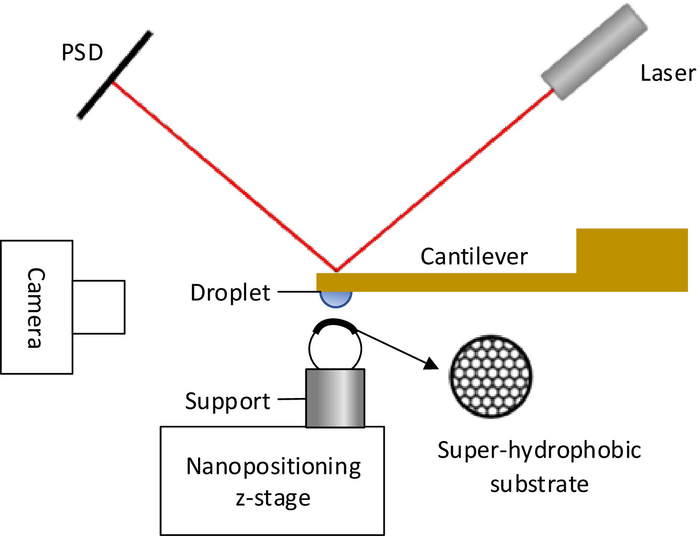Figure 1: The interaction force measurement system. The schematic diagram of the measurement system based on optical lever method for measuring the interaction between droplets and super-hydrophobic substrates. Please click here to view a larger version of this figure.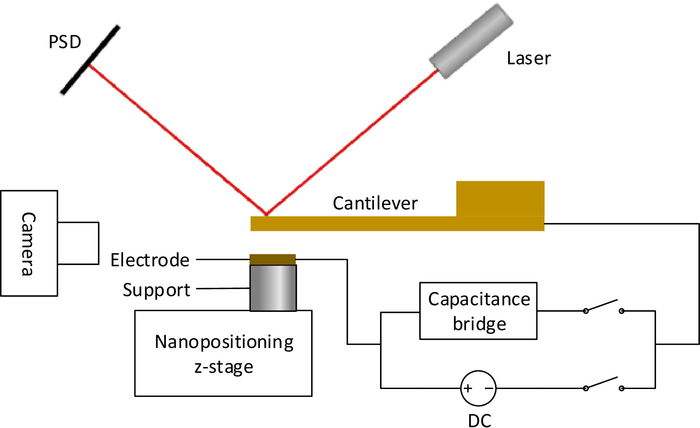Figure 2: The calibration system of SOL. The schematic diagram of the system designed for calibrating the force sensitivity of optical lever using electrostatic force. Please click here to view a larger version of this figure.Figure 3: The dimensions of the millimetric cantilever. Top view and elevation view of the millimetric cantilever. Please click here to view a larger version of this figure.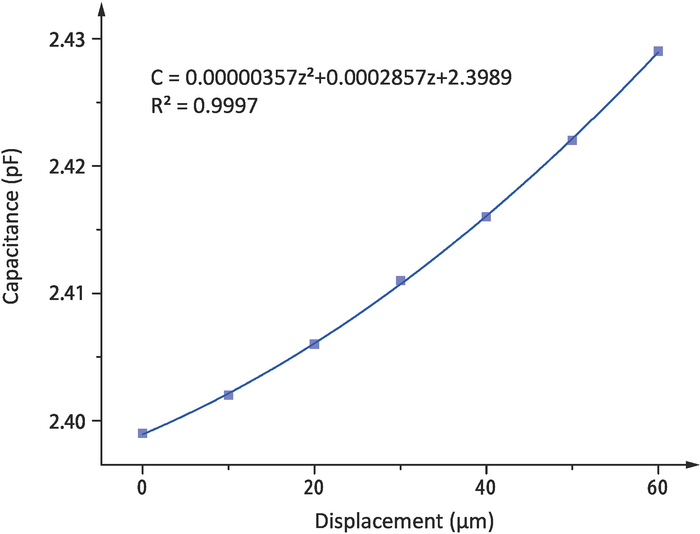Figure 4: The relationship between capacitance and displacement. The quadratic polynomial fitting curve of capacitance C and displacement z in the calibration experiment. Please click here to view a larger version of this figure.Figure 5: The relationship between the output voltage of PSD and the supply voltage. The linear fitting curve of P(1/Vp1-Vp2) and 2(1/Vs12-Vs22) according to Eq. (8). Please click here to view a larger version of this figure.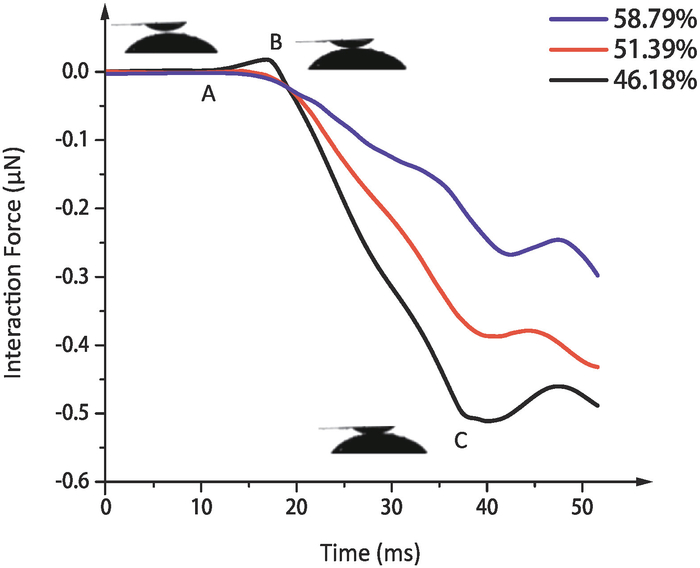Figure 6: The measurement results of interaction force. The interaction forces between droplets and super-hydrophobic substrates with different grid fractions in the air. Different colors represent different grid fractions. Please click here to view a larger version of this figure.Figure 7: Images of three drops of different volumes used in the experiment. The volume of droplets (a), (b), and (c) are 0.0135μL, 0.0087μL, and 0.0073μL respectively. Please click here to view a larger version of this figure.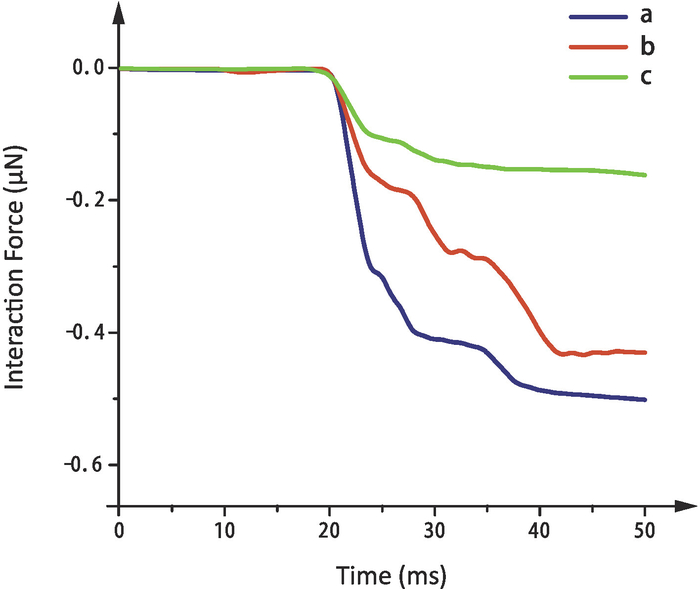Figure 8: Interaction force between the three droplets of different volumes and the substrate. Different colors represent different droplets. Please click here to view a larger version of this figure.

 Time(s) 0 10 20 30 40 50 60 Displacement(μm) 0 10 20 30 40 50 60 Capacitance(pF) 2.399 2.402 2.406 2.411 2.416 2.422 2.429 Time(s) 70 80 90 100 110 120 Displacement(μm) 50 40 30 20 10 0 Capacitance(pF) 2.422 2.416 2.411 2.407 2.403 2.4

Table 1: The calibration results of capacitance gradient. The displacement of the plate electrode and the corresponding capacitance between the cantilever and the electrode in one experiment.

 Time(s) 0 5 10 15 20 25 Supply Voltage(V) 0 25 50 75 100 125 Output voltage of PSD(V) -3.5757 -3.5656 -3.5327 -3.4797 -3.3775 -3.1733 Time(s) 30 35 40 45 50 Supply Voltage(V) 100 75 50 25 0 Output voltage of PSD(V) -3.3765 -3.4786 -3.5321 -3.5644 -3.5755

Table 2: The calibration results of SOL. The supply voltage and the corresponding output voltage of PSD in one experiment.

### Discussion

In this protocol, a measurement system based on optical lever method is assembled and calibrated, which is designed for measuring the interaction force between the droplets and super-hydrophobic substrates. Among the all steps, it is critical to calibrate SOL using electrostatic force. The results of the calibration experiment verify Eq. (8): P(1/Vp1-1/Vp2) is proportional to 2(1/Vs12-1/Vs22) and make it possible to obtain the value of the force to be measured through the output voltage of the PSD. Through the experiment of measuring the interaction force between the droplets and super-hydrophobic substrates of different hydrophobicity, the interaction force decreases with the increase of hydrophobic capacity, which verifies the relationship between hydrophobicity and surface energy of the substrates.

The force measurement method based on a millimetric silicon cantilever is an important complement to the traditional methods. Compared with the high-speed camera method, the optical lever method can accurately measure the force on the nanonewton scale. AFM is usually used to measure the interaction force between micron-scale objects, while the system designed in this paper can be applied on objects of millimeter-scale in the air. This method can be used to measure the force in the scale of sub-micronewtons, and its resolution can reach the scale of nanonewtons.

The method proposed for measuring interaction force in this paper is limited to a small measurement range. A large force will lead to plastic deformation or even break of the silicon cantilever, which will cause incorrect results. In addition, because the principle of this experiment is to measure the interaction force between the droplet and the substrate under the balance of the elastic force of the cantilever and the interaction force, the cantilever can only measure the quasi-static force, but not dynamic force.

The in-depth study of the contact process of droplets and super-hydrophobic structures can help people to improve production efficiency in coating, film, printing and other industrial production. As a generalized adhesion force measurement technique, the substrates in the system can be replaced with substrates made of other materials. For example, a super-hydrophobic substrate with multi-stage microstructures which is made of PDMS (polydimethylsiloxane) can be used. The force measurement system based on optical lever method can also be used in other fields of micro force measurement, such as the interaction force during the coalescence of two droplets and the interaction force between super-hydrophobic substrates and droplets of different surface tension.

### Disclosures

The author has nothing to disclose.

### Acknowledgments

The authors thank the Tianjin Natural Science Foundation (No. 18JCQNJC04800), Tribology Science Fund of State Key Laboratory of Tribology (No. SKLTKF17B18) and National Natural Science Foundation of China (Grant No. 51805367) for their support.

### Materials

 Name Company Catalog Number Comments Camera Shenzhen Andonstar Tech Co., Ltd digital microscope A1 Frame rate: 30 frames/sec; Focal distance: 5 mm - 30 mm Capacitive bridge Andeen-Hagerling AH2550A The capacitive bridge is used to measure the capacitance between the cantilever and the plate electrode. Data acquisition device National Instruments USB-4431 The data acquisition device is used to read the output voltage data. DC power supply Keithley 2410 Voltage range: ±5 μV; Accuracy: 0.012% Grid Electron Microscopy China AGH100, AGH150, AGH300 The grid fractions of AGH100, AGH150 and AGH300 are 46.18%, 51.39% and 58.79% respectively Laser Shenzhen Infrared Laser Technology Co., Ltd. HW650AD100-10BD Laser wavelength: 650 nm Nanoparticle Rust-Oleum 274232 NeverWet Multi-Surface Liquid Repelling Treatment is a revolutionary super hydrophobic coating. Nanopositioning z-stage Physik Instrumente P622.ZCD Travel ranges 50 µm to 250 µm (350 µm open loop); Resolution to 0.1 nm; Linearity error only 0.02% Position sensitive detector Hamamatsu Photonics K.K. S1880 The two-dimensional PSD is used to translate optical signals into electrical signals.